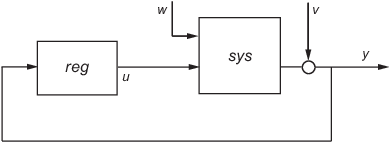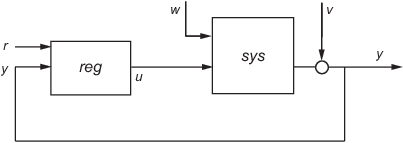Documentation

lqg

Syntax

reg = lqg(sys,QXU,QWV)
reg = lqg(sys,QXU,QWV,QI)
reg = lqg(sys,QXU,QWV,QI,'1dof')
reg = lqg(sys,QXU,QWV,QI,'2dof')
reg = lqg(___,'current')
[reg,info] = lqg(___)

Description

reg = lqg(sys,QXU,QWV) computes an optimal linear-quadratic-Gaussian (LQG) regulator reg given a state-space model sys of the plant and weighting matrices QXU and QWV. The dynamic regulator reg uses the measurements y to generate a control signal u that regulates y around the zero value. Use positive feedback to connect this regulator to the plant output y.The LQG regulator minimizes the cost function

$J=E\left\{\underset{\tau \to \infty }{\mathrm{lim}}\frac{1}{\tau }{\int }_{0}^{\tau }\left[{x}^{T},{u}^{T}\right]{Q}_{xu}\left[\begin{array}{c}x\\ u\end{array}\right]dt\right\}$

subject to the plant equations

$\begin{array}{c}dx/dt=Ax+Bu+w\\ y=Cx+Du+v\end{array}$

where the process noise w and measurement noise v are Gaussian white noises with covariance:

$E\left(\left[\begin{array}{c}w\\ v\end{array}\right]\cdot \left[\begin{array}{cc}w\text{'}& v\text{'}\end{array}\right]\right)=QWV$

reg = lqg(sys,QXU,QWV,QI) uses the setpoint command r and measurements y to generate the control signal u. reg has integral action to ensure that y tracks the command r.The LQG servo-controller minimizes the cost function

$J=E\left\{\underset{\tau \to \infty }{\mathrm{lim}}\frac{1}{\tau }{\int }_{0}^{\tau }\left(\left[{x}^{T},{u}^{T}\right]{Q}_{xu}\left[\begin{array}{c}x\\ u\end{array}\right]+{x}_{i}^{T}{Q}_{i}{x}_{i}\right)\text{ }dt\right\}$

where xi is the integral of the tracking error r - y. For MIMO systems, r, y, and xi must have the same length.

reg = lqg(sys,QXU,QWV,QI,'1dof') computes a one-degree-of-freedom servo controller that takes e = r - y rather than [r ; y] as input.

reg = lqg(sys,QXU,QWV,QI,'2dof') is equivalent to LQG(sys,QXU,QWV,QI) and produces the two-degree-of-freedom servo-controller shown previously.

reg = lqg(___,'current') uses the "current" Kalman estimator, which uses x[n|n] as the state estimate when computing an LQG regulator for a discrete-time system.

[reg,info] = lqg(___) returns the controller and estimator gain matrices in the structure info for any of the previous syntaxes. You can use the controller and estimator gains to, for example, implement the controller in observer form. For more information, see Algorithms.

Examples

Linear-Quadratic-Gaussian (LQG) Regulator and Servo Controller Design

This example shows how to design an linear-quadratic-Gaussian (LQG) regulator, a one-degree-of-freedom LQG servo controller, and a two-degree-of-freedom LQG servo controller for the following system.The plant has three states (x), two control inputs (u), three random inputs (w), one output (y), measurement noise for the output (v), and the following state and measurement equations.

$\begin{array}{l}\frac{dx}{dt}=Ax+Bu+w\\ y=Cx+Du+v\end{array}$

where

$\begin{array}{l}A=\left[\begin{array}{ccc}0& 1& 0\\ 0& 0& 1\\ 1& 0& 0\end{array}\right]\text{ }\text{ }B=\left[\begin{array}{cc}0.3& 1\\ 0& 1\\ -0.3& 0.9\end{array}\right]\\ \\ C=\left[\begin{array}{ccc}1.9& 1.3& 1\end{array}\right]\text{ }D=\left[\begin{array}{cc}0.53& -0.61\end{array}\right]\end{array}$

The system has the following noise covariance data:

$\begin{array}{l}{Q}_{n}=E\left(w{w}^{T}\right)=\left[\begin{array}{ccc}4& 2& 0\\ 2& 1& 0\\ 0& 0& 1\end{array}\right]\\ {R}_{n}=E\left(v{v}^{T}\right)=0.7\end{array}$

For the regulator, use the following cost function to define the tradeoff between regulation performance and control effort:

$J\left(u\right)={\int }_{0}^{\infty }\left(0.1{x}^{T}x+{u}^{T}\left[\begin{array}{cc}1& 0\\ 0& 2\end{array}\right]u\right)dt$

For the servo controllers, use the following cost function to define the tradeoff between tracker performance and control effort:

$J\left(u\right)={\int }_{0}^{\infty }\left(0.1{x}^{T}x+{x}_{i}^{2}+{u}^{T}\left[\begin{array}{cc}1& 0\\ 0& 2\end{array}\right]u\right)dt$

To design the LQG controllers for this system:

1. Create the state-space system by typing the following in the MATLAB Command Window:

A = [0 1 0;0 0 1;1 0 0];
B = [0.3 1;0 1;-0.3 0.9];
C = [1.9 1.3 1];
D = [0.53 -0.61];
sys = ss(A,B,C,D);

2. Define the noise covariance data and the weighting matrices by typing the following commands:

nx = 3;    %Number of states
ny = 1;    %Number of outputs
Qn = [4 2 0; 2 1 0; 0 0 1];
Rn = 0.7;
R = [1 0;0 2]
QXU = blkdiag(0.1*eye(nx),R);
QWV = blkdiag(Qn,Rn);
QI = eye(ny);

3. Form the LQG regulator by typing the following command:

KLQG = lqg(sys,QXU,QWV)
This command returns the following LQG regulator:
A =
x1_e    x2_e    x3_e
x1_e  -6.212  -3.814  -4.136
x2_e  -4.038  -3.196  -1.791
x3_e  -1.418  -1.973  -1.766

B =
y1
x1_e   2.365
x2_e   1.432
x3_e  0.7684

C =
x1_e       x2_e       x3_e
u1   -0.02904  0.0008272     0.0303
u2    -0.7147    -0.7115    -0.7132

D =
y1
u1   0
u2   0

Input groups:
Name        Channels
Measurement       1

Output groups:
Name      Channels
Controls      1,2

Continuous-time model.

4. Form the one-degree-of-freedom LQG servo controller by typing the following command:

KLQG1 = lqg(sys,QXU,QWV,QI,'1dof')
This command returns the following LQG servo controller:
A =
x1_e    x2_e    x3_e     xi1
x1_e  -7.626  -5.068  -4.891  0.9018
x2_e  -5.108  -4.146  -2.362  0.6762
x3_e  -2.121  -2.604  -2.141  0.4088
xi1        0       0       0       0

B =
e1
x1_e   -2.365
x2_e   -1.432
x3_e  -0.7684
xi1         1

C =
x1_e     x2_e     x3_e      xi1
u1  -0.5388  -0.4173  -0.2481   0.5578
u2   -1.492   -1.388   -1.131   0.5869

D =
e1
u1   0
u2   0

Input groups:
Name     Channels
Error       1

Output groups:
Name      Channels
Controls      1,2

Continuous-time model.

5. Form the two-degree-of-freedom LQG servo controller by typing the following command:

KLQG2 = lqg(sys,QXU,QWV,QI,'2dof')
This command returns the following LQG servo controller:
A =
x1_e    x2_e    x3_e     xi1
x1_e  -7.626  -5.068  -4.891  0.9018
x2_e  -5.108  -4.146  -2.362  0.6762
x3_e  -2.121  -2.604  -2.141  0.4088
xi1        0       0       0       0

B =
r1      y1
x1_e       0   2.365
x2_e       0   1.432
x3_e       0  0.7684
xi1        1      -1

C =
x1_e     x2_e     x3_e      xi1
u1  -0.5388  -0.4173  -0.2481   0.5578
u2   -1.492   -1.388   -1.131   0.5869

D =
r1  y1
u1   0   0
u2   0   0

Input groups:
Name        Channels
Setpoint         1
Measurement       2

Output groups:
Name      Channels
Controls      1,2

Continuous-time model.

Algorithms

The controller equations are:

• For continuous time:

$\begin{array}{c}d{x}_{e}=A{x}_{e}+Bu+L\left(y-C{x}_{e}-Du\right)\\ u=-{K}_{x}{x}_{e}-{K}_{i}{x}_{i}\end{array}$

• For discrete time:

$x\left[n+1|n\right]=Ax\left[n|n-1\right]+Bu\left[n\right]+L\left(y\left[n\right]-Cx\left[n|n-1\right]-Du\left[n\right]\right)$

• Delayed estimator:

$u\left[n\right]=-{K}_{x}x\left[n|n-1\right]-{K}_{i}{x}_{i}\left[n\right]$

• Current estimator:

$\begin{array}{c}u\left[n\right]=-{K}_{x}x\left[n|n\right]-{K}_{i}{x}_{i}\left[n\right]-{K}_{w}w\left[n|n\right]\\ =-{K}_{x}x\left[n|n-1\right]-{K}_{i}{x}_{i}\left[n\right]-\left({K}_{x}{M}_{x}+{K}_{w}{M}_{w}\right){y}_{inn}\left[n\right]\end{array}$

${y}_{inn}\left[n\right]=y\left[n\right]-Cx\left[n|n-1\right]-Du\left[n\right]$

Here,

• A, B, C, and D are the state-space matrices of the LQG regulator, reg.

• xi is the integral of the tracking error r - y.

• Kx, Kw, Ki, L, Mx, and Mw are the controller and estimator gain matrices returned in info.